# How to trim to i.e. 2 decimal places in the Data window?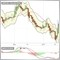277

in Indicator Data window,the numbers (after dot) show bunch of zeroes: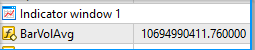i need to be shown there only 2 decimals after dot.

used this function, but still doesnt help, there are still ZEROes shown...

double RoundNumber(double number, int digits) {  number = MathRound(number * MathPow(10, digits));  return (number * MathPow(10, -digits)); }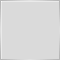Moderator
2315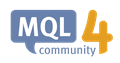IndicatorDigits - Custom Indicators - MQL4 Reference
• docs.mql4.com
IndicatorDigits - Custom Indicators - MQL4 Reference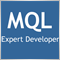1623

I use NormalizeDouble() quite often.

https://www.mql5.com/en/docs/convert/normalizedouble

Example:

```double something = 0.123456789;
double someother = NormalizeDouble(something,2);
Comment("Something = "+(string)something+"\nSomeother: "+(string)someother);```

The output looks like this: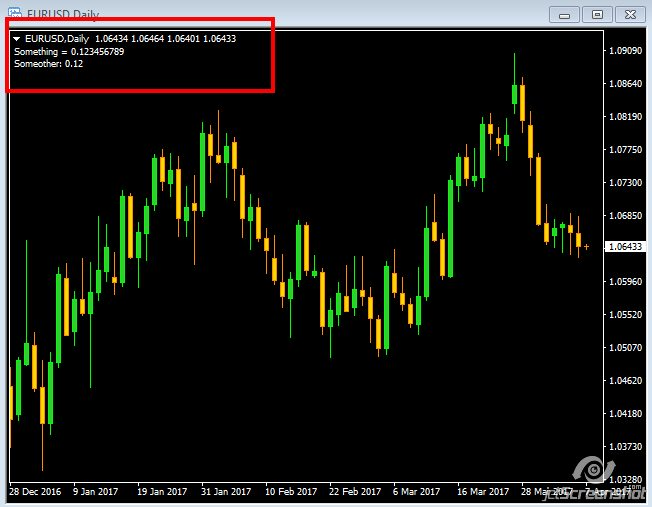As with most thing in life, there is more than one way to accomplish the desired results.Documentation on MQL5: Conversion Functions / NormalizeDouble
• www.mql5.com
Conversion Functions / NormalizeDouble - Reference on algorithmic/automated trading language for MetaTrader 5Moderator
2315

Both those methods (MathRound and NormalizeDouble) do not change the output of the data window. For that, you have to use IndicatorDigits() for MQL4 or IndicatorSetInteger(INDICATOR_DIGITS,x) for both MQL4 and MQL55

hi i am having a problem , i get the reading of my indicator in the data window in  integers like 1,2 ,-1,-2 and i need the readings to be in decimal like 1.2133 , 2.5463 soo please help meModerator
30417

68659133:
hi i am having a problem , i get the reading of my indicator in the data window in  integers like 1,2 ,-1,-2 and i need the readings to be in decimal like 1.2133 , 2.5463 soo please help me

Answer is in the post above yours - didn't you read the topic?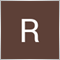161

Had a similar issue. All methods still had some results with rounding errors so I arrived at the following: convert the number to a string and truncate.

```string TruncateNumber(string number, int decimalPoints=2)
{
int start_index = StringFind(number, ".");
if (start_index == -1) return number;

string vals = {"", ""};
StringSplit(number, '.', vals);

if (StringLen(vals) <= decimalPoints ) return number;

return StringConcatenate(vals, ".", StringSubstr(vals, 0, 2));
}```Moderator
30417

Rene K Serulle:

Had a similar issue. All methods still had some results with rounding errors so I arrived at the following: convert the number to a string and truncate.15

source:

symb_pos_diff_lots = 0.0800000000001;

//-------

code:

symb_pos_diff_lots = (MathFloor(symb_pos_diff_lots * 100)) / 100;
symb_pos_diff_lots = NormalizeDouble(symb_pos_diff_lots, 2);

//-------

result:

symb_pos_diff_lots = 0.08;

//-------

P.S. Multiply your number with * 100, Round it in your way .. and then devide to 100 again ..

then -> NormalizeDouble ;)Moderator
30417

dzfx5:15

Keith Watford: